: The Digit Sum Process. : Curious Properties of Reiterated : The Collatz Problem

# 2. The Digits Cube Sum Problem.

We are going to study the DCS (digit cube sum) problem.

Definition 2.1.   We define the dcs function by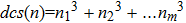, where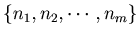is the list of the digits of an integer n.

If we apply dcs(n) function to a natural number n repeatedly, then we get the sequence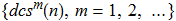. For the detail of the theory of the DCS problem see .

Example 2.1.   For Example, we start with n= 12. Then dcs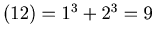, dcs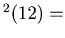dcs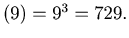dcs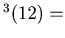dcs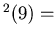dcsWe continue this operation and we get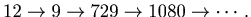Lemma 2.1.   We have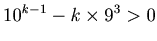(2.1)

for natural number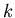such that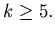Proof.   Let, then we have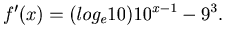(2.2)

When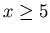,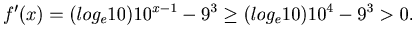(2.3)

Since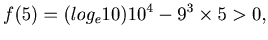(2.4)

by 2.3 we have 2.1.

Theorem 1.   If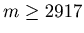, then dcs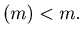Proof.   Suppose that m has k digits, then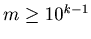. Since each digit is less than or equal to 9, dcs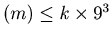. By Lemma 2.1 for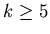we have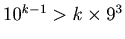, and hence dcsIfand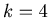, then dcs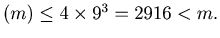Theorem 1 has been already proved in p.27 of.
Theorem 2.   For any natural number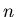the sequence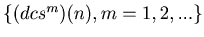eventually will enter into a loop. In other words there exist numbers p and q such that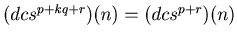for any natural number k and any integer r such that, where we call q the length of the loop. The sequence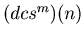enters into a loop when m = p.
Proof.   If we start with a natural number n, we have a sequenceby applying the function dcs to the number n repeatedly. When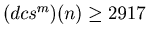, by Theorem 1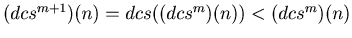. If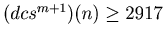, by Theorem 1 we have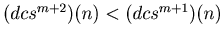. In this way this sequence decreases while the value of the sequence is bigger or equal to 2917. Therefore there exists a natural number t such that. In this way we can prove that there are infinite number of m such that, and hence there is a non-negative integer u such that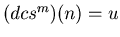for at least two values of m. Let v be the smallest integer such thatand let w be the second smallest integer such that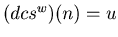.
Then clearly,...,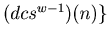is a loop.
Example 2.2.   If we apply dcs function to the number 115 for 20 times, then we get the sequence
{115, 127, 352, 160, 217, 352, 160, 217, 352, 160, 217, 352, 160, 217, 352, 160, 217, 352, 160, 217, 352}. From this sequence we get Graph 2.1.
Graph 2.1.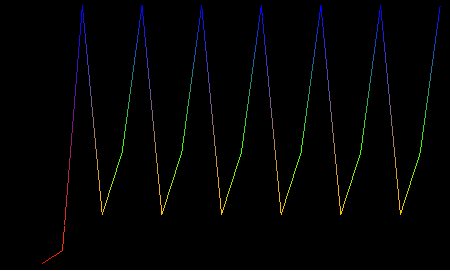In Example 2.2 the dcs function produces a sequence with a loop. By Theorem 2 successive application of dcs function will produce a sequence with a loop. Now we are going to look for all the loops. By Theorem 1 we have only to look for loops of the sequences that start with a number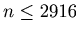, and we can find loops by calculation of computers.
Example 2.3.   If we use the Mathematica function in Example 10.3, we can find all the loops. The output of the program is
{{1}, {55, 250, 133}, {133, 55, 250}, {136, 244}, {153}, {160, 217, 352}, {217, 352, 160}, {244, 136}, {250, 133, 55}, {352, 160, 217}, {370}, {371}, {407}, {919, 1459}, {1459, 919}}.
Loops of the length of 3 are {55, 250, 133},{160, 217, 352}, and loops of the length of 2 are {136, 244}, {919, 1459}. Fixed points are {1}, {153}, {370}, {371}, {407}.: The Digit Sum Process. : Curious Properties of Reiterated : The Collatz Problem